Question

Q: What is the pH of a solution containing 0.125 M KH2PO4 and 0.175 K2HPO4 Ka (H2PO4-) = 6.2 x 10-8 Ka (HPO42-) = 4.8 x 10-13

Q: A 0.15 M solution of a weak acid is 3.0 % dissociated. What is the Ka of this acid?

Q: A solution of aspirin was prepared that is 0.16 M. The pH of this solution was measured to be 2.43. What is the Ka of aspirin?

Q: A solution of formic acid (Ka = 1.8 x 10-4). The pH is 2.8. What is the initial concentration of formic acid.

Q: If the pH of a 1.7 x 10-3 M solution of codeine, a weak base, is 9.59, what is the Kb of this drug?

Q: What mass of sodium acetate must be added to 750 mL of 0.200 M CH3COOH (Ka = 1.8 x10-5) to generate a buffer of pH = 5.0

Q: What mass of NaOH must be added to 750.0 mL of 0.200 M CH3COOH to generate a buffer of pH = 5.0.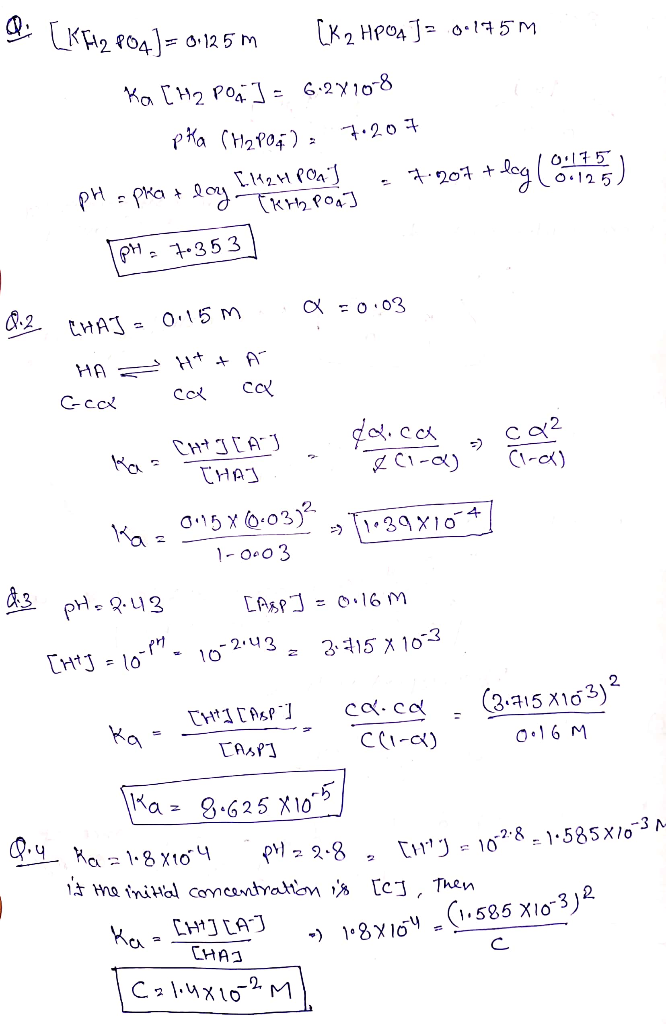#### Earn Coins

Coins can be redeemed for fabulous gifts.

Similar Homework Help Questions
• ### A buffer solution is 0.451 M in KH2PO4 and 0.335 M in K2HPO4. If Ka for...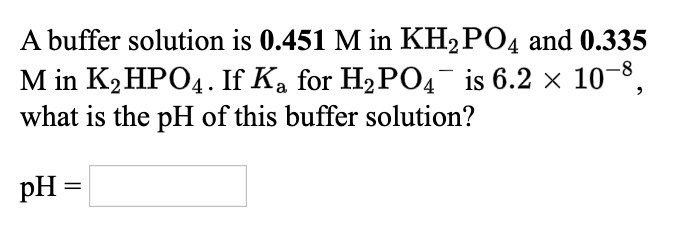A buffer solution is 0.451 M in KH2PO4 and 0.335 M in K2HPO4. If Ka for H2PO4- is 6.2 x 10^-8 , what is the pH of this buffer solution? A buffer solution is 0.451 M in KH P04 and 0.335 M in K2HPO4. If Ką for H2PO4 is 6.2 x 10-8, what is the pH of this buffer solution? pH =

• ### What is the pH of a buffer solution containing 0.13 M HF and 5.0×10−2 M NaF?...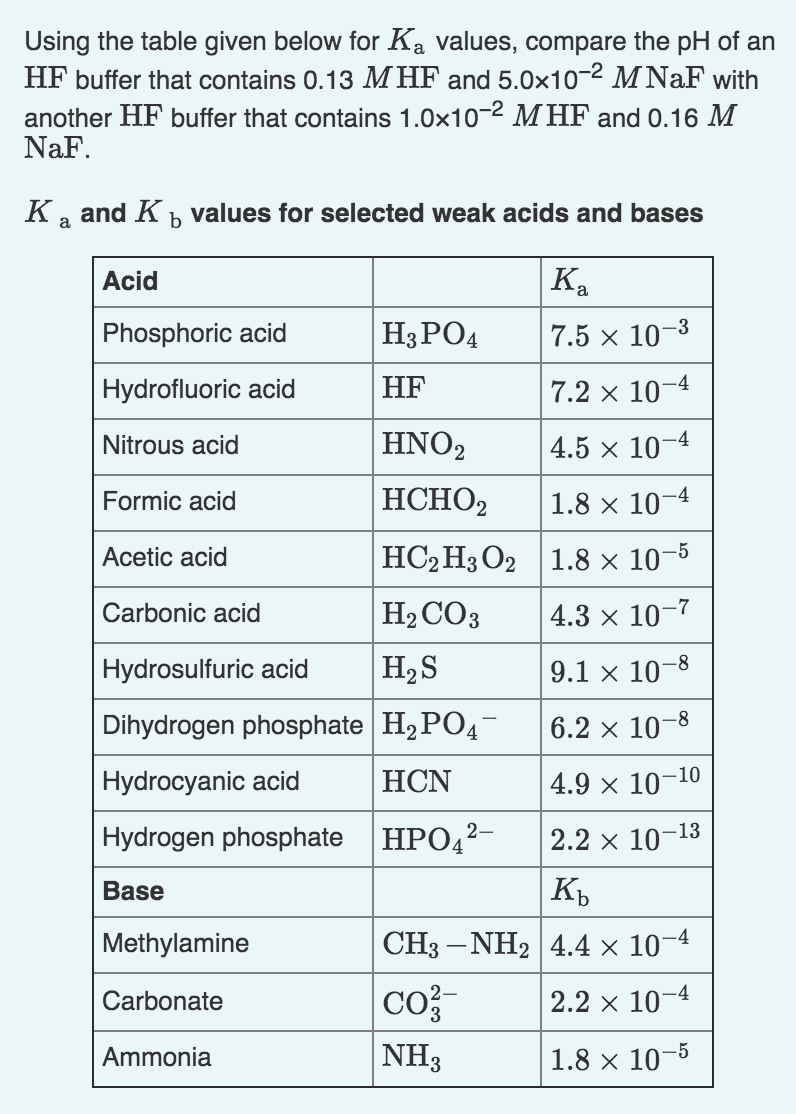What is the pH of a buffer solution containing 0.13 M HF and 5.0×10−2 M NaF? What is the pH of a buffer solution containing 1.0×10−2 M HF and 0.16 M NaF? Using the table given below for Ka values, compare the pH of an HF buffer that contains 0.13 MHF and 5.0x10-2 M NaF with another HF buffer that contains 1.0x10-2 MHF and 0.16 M NaF. Ka and K b values for selected weak acids and bases HF Acid...

• ### 6. What is the pH of a buffered system made by dissolving 17.42 g of KH2PO4 and 20.41 g of K2HPO4 in water to give...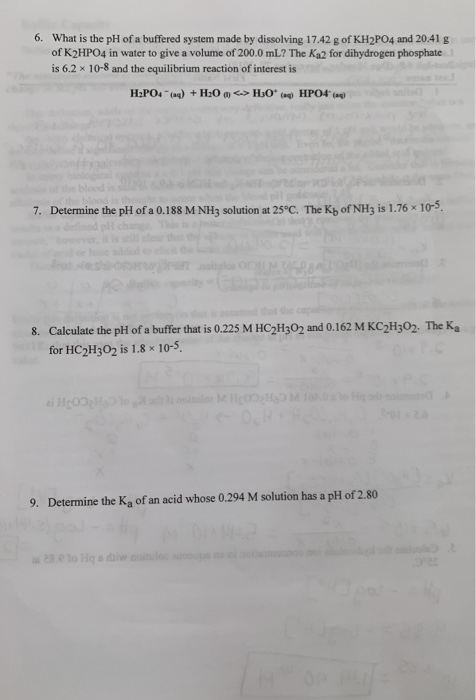6. What is the pH of a buffered system made by dissolving 17.42 g of KH2PO4 and 20.41 g of K2HPO4 in water to give a volume of 200.0 mL? The Ka2 for dihydrogen phosphate is 6.2 x 10-8 and the equilibrium reaction of interest is H2PO4 (4) + H20 <-> H30* ( HPO4 (4) 7. Determine the pH of a 0.188 M NH3 solution at 25°C. The Kh of NH3 is 1.76 x 10-5. 8. Calculate the pH of...

• ### Determine the [H3O*1 and pH of a 0.200 M formic acid (HCHO2) solution. The Ka of...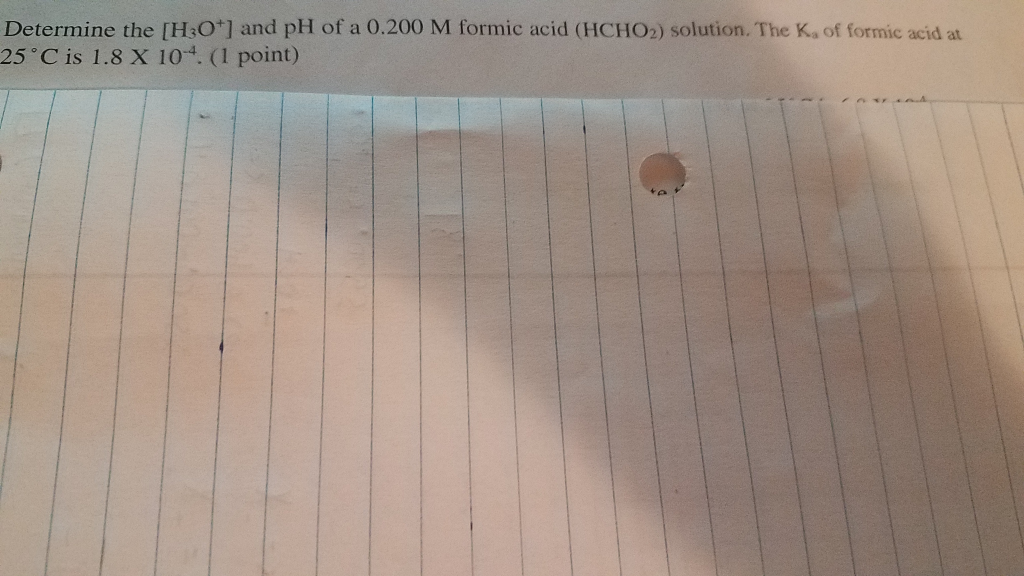Determine the [H3O*1 and pH of a 0.200 M formic acid (HCHO2) solution. The Ka of formic acid at 25 °C is 1.8 X 10. (1 point)

• ### Henderson-Hasselbach equation: pH- pKa log (IA-|/IHA]) 1. Phosphate buffer is a mixture of KH2PO4 and K2HPO4....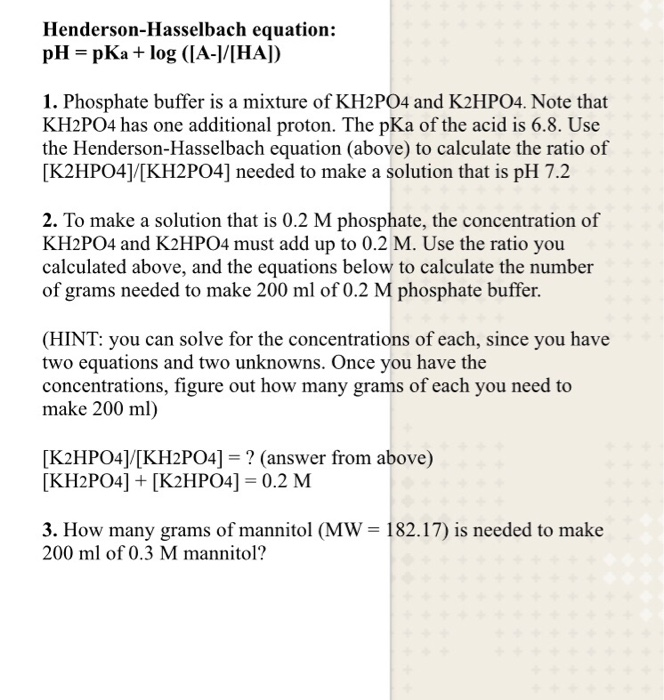Henderson-Hasselbach equation: pH- pKa log (IA-|/IHA]) 1. Phosphate buffer is a mixture of KH2PO4 and K2HPO4. Note that KH2PO4 has one additional proton. The pKa of the acid is 6.8. Use the Henderson-Hasselbach equation (above) to calculate the ratio of [K2HPO41[KH2PO4] needed to make a solution that is pH 7.2 2. To make a solution that is 0.2 M phosphate, the concentration of KH2PO4 and K2HPO4 must add up to 0.2 M. Use the ratio you calculated above, and the...

• ### A buffer solution is 0.409 M in H3PO4 and 0.258 M in KH2PO4. If Kal for...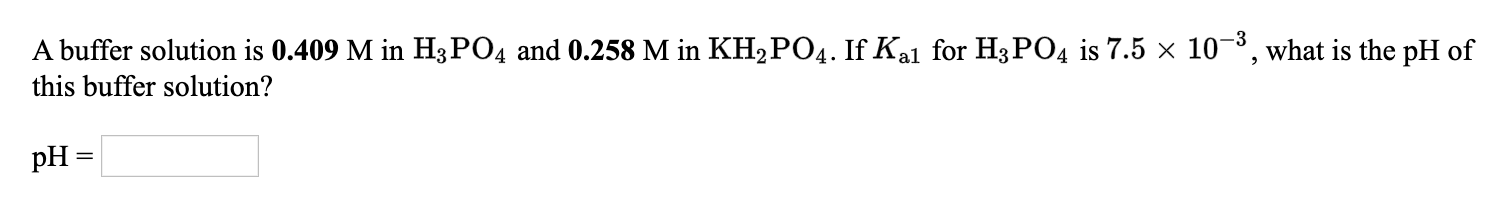A buffer solution is 0.409 M in H3PO4 and 0.258 M in KH2PO4. If Kal for H3PO4 is 7.5 x 10-3, what is the pH of this buffer solution? pH = A buffer solution is 0.407 M in CH3COOH and 0.331 M in CH3COONa . If K, for CH3COOH is 1.8x10-5, what is the pH of this buffer solution?

• ### 1.555 24. For a solution of 0.25 M HC1, determine the (H O'1, (oH-1, and pH....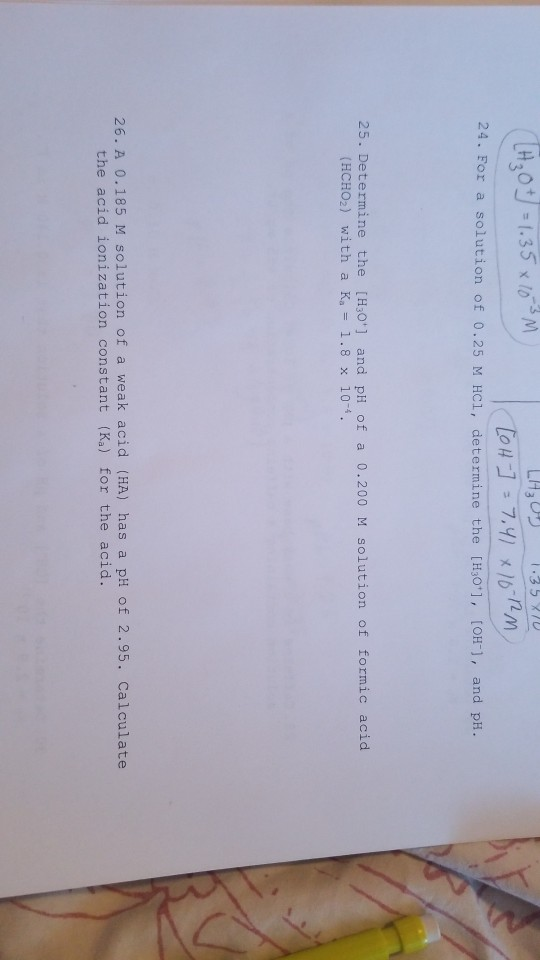1.555 24. For a solution of 0.25 M HC1, determine the (H O'1, (oH-1, and pH. LH3ひっ 35 3ID 25. Determine the [H3o] and pH of a 0.200 M solution of formic acid (HCHOz) with a Ka= 1.8 x 10-4 . 26. A 0.185 M solution of a weak acid (HA) has a pH of 2.95. Calculate the acid ionization constant (Ka) for the acid.

• ### A microbiologist is preparing a medium on which to culture E. coli bacteria. She buffers the medium at pH 7.00 to minimi...

A microbiologist is preparing a medium on which to culture E. coli bacteria. She buffers the medium at pH 7.00 to minimize the effect of acid-producing fermentation. What volumes of equimolar aqueous solutions of K2HPO4 and KH2PO4 must she combine to make 750.0 mL of the pH 7.00 buffer? Ka values for phosphoric acid: Ka1 = 7.2 ×10−3 Ka2 = 6.3 ×10−8 Ka3 = 4.2 × 10−13 Volume H2PO4− = ??? mL Volume HPO42− = ??? mL

• ### 1.) a buffer solution is 0.453 M im HNO2 and 0.339 M in NaNO2. If Ka...

1.) a buffer solution is 0.453 M im HNO2 and 0.339 M in NaNO2. If Ka for HNO2 is 4.5x10^-4, what is the pH of this buffer solution? pH = ???? 2.) A buffer solution is 0.373 M in H2C2O4 and 0.303 M in KHC2O4. If Ka1 for H2C2O4 is 5.9x10^-2, what is the pH of this buffer solution? pH = ???? 3.) a buffer solution is 0.333 M in KH2PO4 and 0.248 M in K2HPO4. If Ka for H2PO4^-...

• ### Calculate the pH of a buffer solution containing 0.100 M CH3COOH and 0.100 M CH3COONa ;...

Calculate the pH of a buffer solution containing 0.100 M CH3COOH and 0.100 M CH3COONa ; Ka of CH3COOH = 1.8 x 10-5